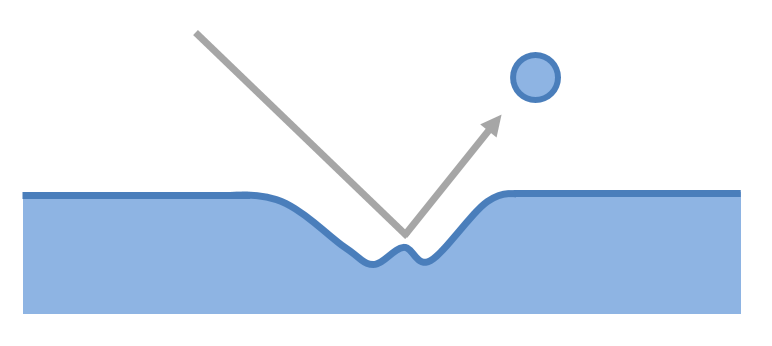# Summary

The flow of a gas-liquid multiphase system may cause erosion if velocities are high. This article presents an empirical relationship for estimating whether erosion will occur in a system at a certain velocity.

# Definitions

 : Cross sectional area of flow : Erosional constant : mass flux : Mass flow rate : Gas to liquid ratio in metric units (volume per volume) : Gas to liquid ratio in traditional units : Specific gravity : Velocity : Density

Subscripts:

 : Phase 1 : Phase 2 : Average

# Introduction

In multiphase systems high fluid velocities are often desirable to decrease the chances of slug flow or particle settling. High velocities, however will increase the energy with which liquid droplets impact on pipe and vessel walls, which, if they may have sufficient force will cause erosion or accelerate corrosion.# Estimating Erosion

## Erosion Velocity

The velocity at which erosion may start to occur can be calculated using the average density and velocity of the flow and the following empirical equation:

Calculation of the components of this equation will be presented in the following sections.

## Erosional Constant

API 14E provides the following typical values for the empirical erosional constant based on industrial experience.

Conditions
Solids-free, continuous service100
Solids-free, intermittent service125
Corrosion resistant alloys or corrosion inhibitor, continuous service150-200
Corrosion resistant alloys or corrosion inhibitor, intermittent service250

## Average Density

The average density of the gas-liquid multiphase system can be calculated one either a mass or volumetric basis, depending on the information available:

If the mass flow rates of each phase are known the following equation may be used:

Where the mass flux is equal to the ratio of mass flow rate of a phase to the flow area .

If the gas/liquid ratio is known the following equation may be used:

The gas to liquid ratio can be converted from traditional to metric units as follows:

1. Chemical Engineering Volume 1, Sixth Edition: Fluid Flow, Heat Transfer and Mass Transfer (Coulson and Richardsons Chemical Engineering)